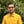Trusted answers to developer questions
Trusted Answers to Developer Questions

Related Tags

scala
math
sin
communitycreator

# What is Math.sin() in Scala?Harris Amjad

Grokking Modern System Design Interview for Engineers & Managers

Ace your System Design Interview and take your career to the next level. Learn to handle the design of applications like Netflix, Quora, Facebook, Uber, and many more in a 45-min interview. Learn the RESHADED framework for architecting web-scale applications by determining requirements, constraints, and assumptions before diving into a step-by-step design process.

The sin() function in Scala returns the sine of a number in the radians. The illustration below shows the mathematical representation of the sin() function.

Mathematical representation of the sin() function
• The scala.math._ header file is required for this function.
• This sin() function only works for right-angled triangles.

### Syntax

def sin(x: Double): Double


### Parameter

This function requires a number that represents an angle in radians as a parameter.

In order to convert degrees to radians, use the following formula:

radians = degrees * ( Pi / 180.0 )


### Return value

sin() returns the sine of a number in radians which is sent as a parameter.

If the parameter value is NaN, positive infinity, or negative infinity, then it returns NaN.

### Code

import scala.math._object Main extends App {    //positive number in radians    println(s"The value of sin(2.3) = ${sin(2.3)}"); // negative number in radians println(s"The value of sin(-2.3) =${sin(-2.3)}");      //converting the degrees angle into radians and then applying sin()    // degrees = 45.0    // PI = 3.14159265    // result first converts degrees to radians then apply sin    println(s"The value of sin(45.0) = ${sin(45.0 * (Pi / 180.0))}"); //error outputs println(s"The value of sin(Double.PositiveInfinity) =${sin(Double.PositiveInfinity)}");    println(s"The value of sin(Double.NegativeInfinity) = ${sin(Double.NegativeInfinity)}"); println(s"The value of sin(Double.NaN) =${sin(Double.NaN)}");        }

RELATED TAGS

scala
math
sin
communitycreator

CONTRIBUTORHarris Amjad

Grokking Modern System Design Interview for Engineers & Managers

Ace your System Design Interview and take your career to the next level. Learn to handle the design of applications like Netflix, Quora, Facebook, Uber, and many more in a 45-min interview. Learn the RESHADED framework for architecting web-scale applications by determining requirements, constraints, and assumptions before diving into a step-by-step design process.

Keep Exploring

Learn in-demand tech skills in half the time